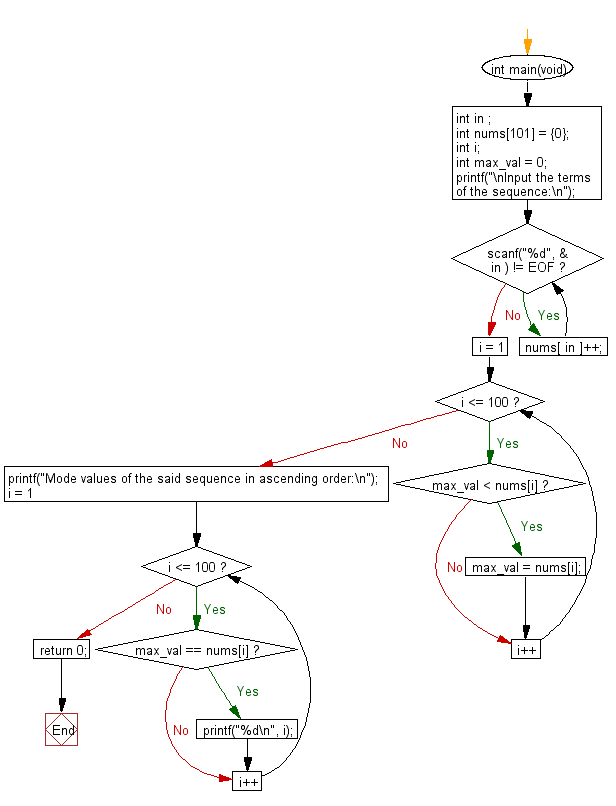﻿ C Program: Find the most frequent element(s) in a sequence

# C Exercises: Find the element(s) which occurs most frequently in a given sequence

## C Basic Declarations and Expressions: Exercise-140 with Solution

Write a C program that reads a sequence of integers and finds the element that occurs most frequently.

Sample Solution:

C Code:

``````#include <stdio.h>
#include<stdlib.h>
#include<math.h>
#include<string.h>

int main(void) {
int in;
int nums = {0}; // Initialize an array to store frequency of each number (from 1 to 100)
int i;
int max_val = 0; // Variable to keep track of the maximum frequency

printf("\nInput the terms of the sequence:\n");

while (scanf("%d", &in) != EOF) // Keep reading numbers until end of input (EOF)
nums[in]++; // Increment the frequency count for the input number

// Find the maximum frequency
for (i = 1; i <= 100; i++) {
if (max_val < nums[i])
max_val = nums[i];
}

printf("Mode values of the said sequence in ascending order:\n");

// Print numbers that have the maximum frequency
for (i = 1; i <= 100; i++) {
if (max_val == nums[i])
printf("%d\n", i);
}

return 0; // End of the program
}
``````

Sample Output:

```
Input the terms of the sequence:
5
2
4
6
8
10
^Z
Mode values of the said sequence in ascending order:
2
4
5
6
8
10
```

Flowchart:C programming Code Editor:

What is the difficulty level of this exercise?

Test your Programming skills with w3resource's quiz.

﻿